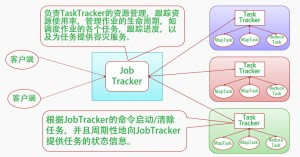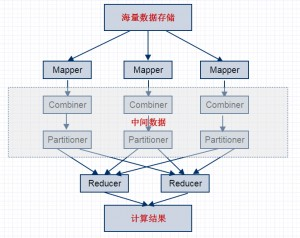MapReduce并行计算流程介绍

3625℃• 当你向MapReduce框架提交一个计算作业时，它会首先把计算作业拆分成若干个Map任务，然后分配到不同的节点上去执行。
• 每个Map节点将输入的键值对(k1;v1)处理为[(k2;v2)]表示的一组中间数据。中间数据进行合并(Combiner)处理，[(k2;v2)]中相同主键下的不同数值合并到一个列表[v2]中，为(k2;[v2])
• 等待所有的Map节点任务完成，对所有的中间数据使用一定的策略进行划分(Partitioner)处理，保证相关主键下的数据发送到同一个Reduce节点。
• 再由Reduce节点将输入(k2;[v2])，整理或进一步处理，输出[(k3; v3)]
• 最后把结果进行合并输出最终结果。``````
hello a
hello b
``````

``````

(0,"hello a")
(8,"hello b")
map(key,value,context) {
String[] words = value.split("\t");
for(String word : words) {
context.write(word,1);
}
}

[(hello,1),(a,1)]
[(hello,1),(b,1)]
``````

reduce阶段（分组排序）：

``````

(a,1)
(b,1)
(hello,[1,1])
reduce(key,value,context) {
String word = key;
int sum = 0;
for(int i : value) {
sum += i;
}
context.write(word,sum);
}

(a,1)
(b,1)
(hello,2)``````